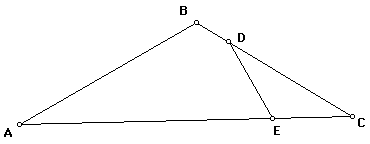# Similar TrianglesTriangles DEC and ABC have three pairs of angles equal in measure. If angle DEC is greater than angle EDC, then write as many possible proportions comparing the side lengths of the two triangles.Extension:
What if you start out with a triangle that has three pairs of equal proportions among the side lengths. What can you conclude about the angle measures?